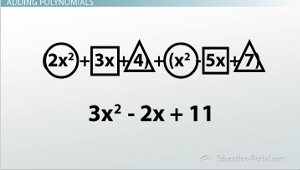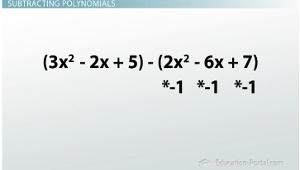# How to Add, Subtract and Multiply Polynomials

An error occurred trying to load this video.

Try refreshing the page, or contact customer support.

Coming up next: How to Divide Polynomials with Long Division

### You're on a roll. Keep up the good work!

Replay
Your next lesson will play in 10 seconds
• 0:04 Adding Polynomials
• 2:19 Subtracting Polynomials
• 4:07 Multiplying Polynomials
• 6:18 Lesson Summary

Want to watch this again later?

Timeline
Autoplay
Autoplay
Speed

#### Recommended Lessons and Courses for You

Lesson Transcript
Instructor: Kathryn Maloney

Kathryn teaches college math. She holds a master's degree in Learning and Technology.

Adding, subtracting and multiplying polynomials are, basically, the same as adding, subtracting and multiplying numbers. They only difference is that we have a pesky variable to worry about, but this video will show you that's no problem, so no worries! This method has worked for many of my students, and I think it will work for you, too!Adding polynomials is very easy! There are many ways to add polynomials that have been taught, but here's my favorite. I look at the problem and put a circle, square or triangle around the like terms so I don't get them confused.

Let's look at this example.

(2x^2 + 3x + 4) + (x^2 - 5x + 7)

I like to start from the left. I circle 2x^2. Now, I look for another like term to 2x^2. Of course, that's x^2, so I circle it.

Then I add them: 2x^2 + x^2 is 3x^2, and then I write that down as part of my answer.

The next term is 3x. I put a square around that one. Then look for another one. Of course, we have -5x, so I put a square around that one too.

Then add them: 3x + -5x is -2x, and I write that down as the next part of my answer.

You always want to double check that there aren't any more.

Finally, we have 4. I continue the same idea, but I put a triangle around it. Now I look for another like term to 4. Of course, that's 7, so I put a triangle around that one.

Then I add them: 4 + 7 is 11, and I write that down as the last part of my answer.

It's always good to double check that you have everything in a circle, square or triangle. When you get longer polynomials, it's easy to miss terms!

So here's my final answer: 3x^2 - 2x + 11.

'Okay, Kathryn, do I have to use circles, squares and triangles?' No! If you're good at adding polynomials, you can cross off as you go, but for those that haven't had enough practice, this is definitely my suggestion.

'Kathryn, what about problems that have more terms? What other shapes or ideas do you have?' Great question! You can use different colors to circle like terms. I don't use it here for the benefit of students who are color blind, but you could have used red to circle the x^2s, blue to circle the xs and green for the numbers. I've also used the method of underlining like terms too! Whatever you choose is how you will distinguish the different terms!## Subtracting Polynomials

In subtraction, let me show you the underlining method.

(3x^2 - 2x + 5) - (2x^2 - 6x + 7)

First, I am going to distribute the -1 into the second expression. That will make this an addition problem!

The first expression stays the same: 3x^2 - 2x + 5. We will distribute the negative, like this: -1 * 2x^2, which is -2x^2; -1 * -6x, which is a positive 6x; and -1 * 7, which is -7. This gives us our new subtraction problem: (3x^2 - 2x + 5) + (-2x^2 + 6x - 7).

Remember, I am going to show you the method of underlining instead of circling to add the expressions.

We look at the first term 3x^2 and underline it. Now, I continue to look for a like term. Here it is, -2x^2, and I underline it. Now I add them: 3x^2 + (-2x^2), and we get x^2. That's going to be the first term of our answer.

The second term is -2x, and this time, I put a squiggly line under it. Now I continue to look for a like term. Here it is: 6x, and I put a squiggly line under that one. I add -2x + 6x, and I get 4x. That's the second term in our answer.

To unlock this lesson you must be a Study.com Member.

### Register to view this lesson

Are you a student or a teacher?

### Unlock Your Education

#### See for yourself why 30 million people use Study.com

##### Become a Study.com member and start learning now.
Back
What teachers are saying about Study.com

### Earning College Credit

Did you know… We have over 160 college courses that prepare you to earn credit by exam that is accepted by over 1,500 colleges and universities. You can test out of the first two years of college and save thousands off your degree. Anyone can earn credit-by-exam regardless of age or education level.